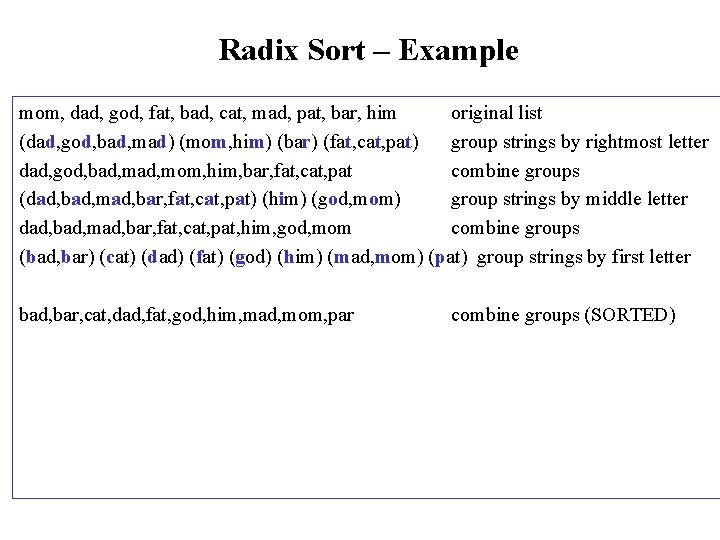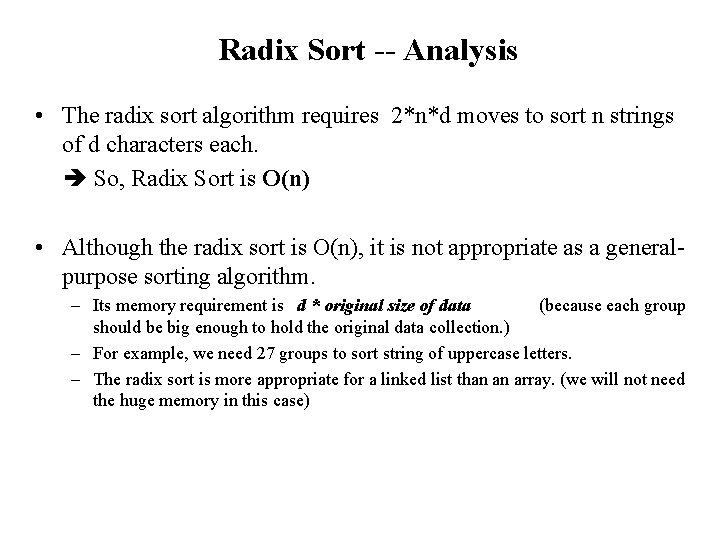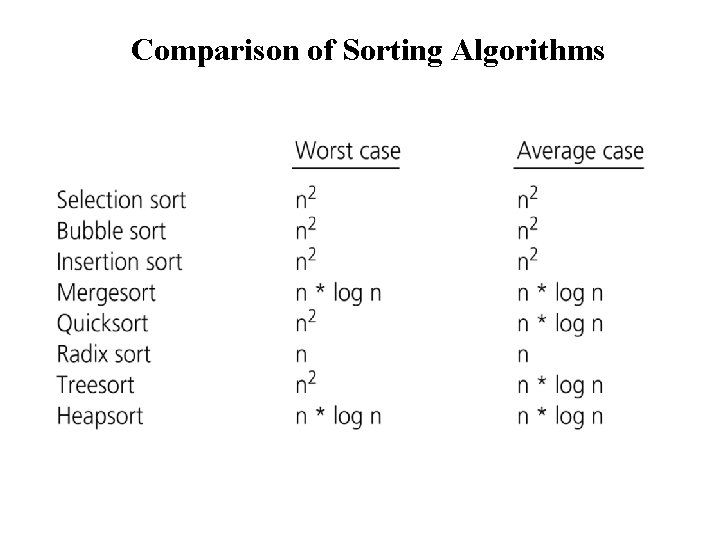# Sorting Algorithms Sorting Sorting is a process that

• Slides: 53Sorting AlgorithmsSorting • Sorting is a process that organizes a collection of data into either ascending or descending order. • An internal sort requires that the collection of data fit entirely in the computer’s main memory. • We can use an external sort when the collection of data cannot fit in the computer’s main memory all at once but must reside in secondary storage such as on a disk. • We will analyze only internal sorting algorithms. • Any significant amount of computer output is generally arranged in some sorted order so that it can be interpreted. • Sorting also has indirect uses. An initial sort of the data can significantly enhance the performance of an algorithm. • Majority of programming projects use a sort somewhere, and in many cases, the sorting cost determines the running time. • A comparison-based sorting algorithm makes ordering decisions only on the basis of comparisons.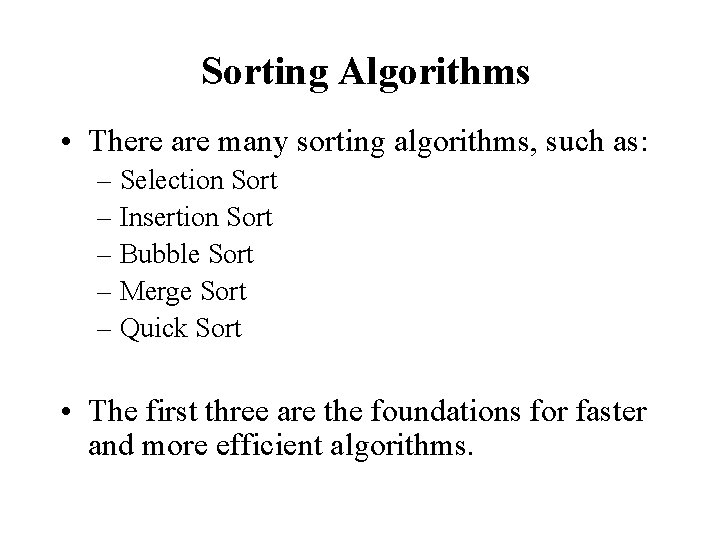Sorting Algorithms • There are many sorting algorithms, such as: – Selection Sort – Insertion Sort – Bubble Sort – Merge Sort – Quick Sort • The first three are the foundations for faster and more efficient algorithms.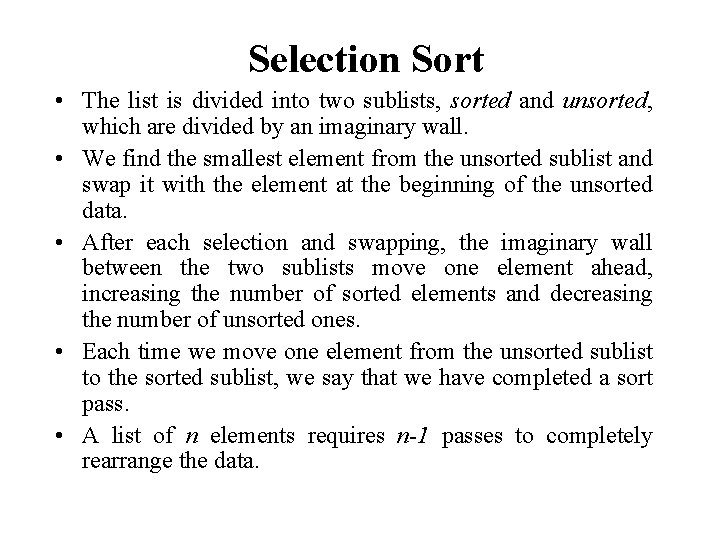Selection Sort • The list is divided into two sublists, sorted and unsorted, which are divided by an imaginary wall. • We find the smallest element from the unsorted sublist and swap it with the element at the beginning of the unsorted data. • After each selection and swapping, the imaginary wall between the two sublists move one element ahead, increasing the number of sorted elements and decreasing the number of unsorted ones. • Each time we move one element from the unsorted sublist to the sorted sublist, we say that we have completed a sort pass. • A list of n elements requires n-1 passes to completely rearrange the data.Sorted Unsorted 23 78 45 8 32 56 Original List 8 78 45 23 32 56 After pass 1 8 23 45 78 32 56 After pass 2 8 23 32 78 45 56 After pass 3 8 23 32 45 78 56 After pass 4 8 23 32 45 56 78 After pass 5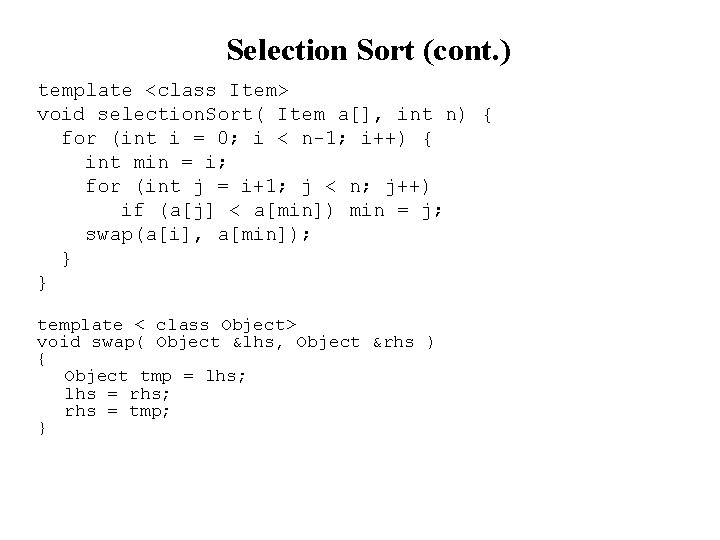Selection Sort (cont. ) template <class Item> void selection. Sort( Item a[], int n) { for (int i = 0; i < n-1; i++) { int min = i; for (int j = i+1; j < n; j++) if (a[j] < a[min]) min = j; swap(a[i], a[min]); } } template < class Object> void swap( Object &lhs, Object &rhs ) { Object tmp = lhs; lhs = rhs; rhs = tmp; }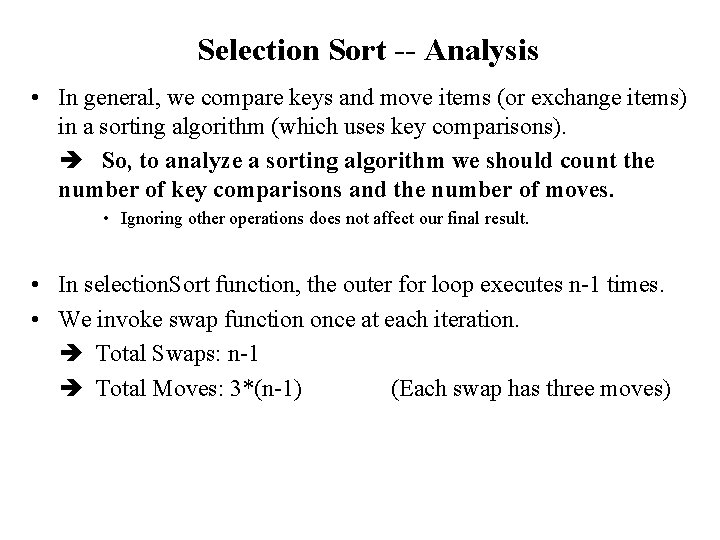Selection Sort -- Analysis • In general, we compare keys and move items (or exchange items) in a sorting algorithm (which uses key comparisons). So, to analyze a sorting algorithm we should count the number of key comparisons and the number of moves. • Ignoring other operations does not affect our final result. • In selection. Sort function, the outer for loop executes n-1 times. • We invoke swap function once at each iteration. Total Swaps: n-1 Total Moves: 3*(n-1) (Each swap has three moves)Selection Sort – Analysis (cont. ) • The inner for loop executes the size of the unsorted part minus 1 (from 1 to n-1), and in each iteration we make one key comparison. # of key comparisons = 1+2+. . . +n-1 = n*(n-1)/2 So, Selection sort is O(n 2) • The best case, the worst case, and the average case of the selection sort algorithm are same. all of them are O(n 2) – This means that the behavior of the selection sort algorithm does not depend on the initial organization of data. – Since O(n 2) grows so rapidly, the selection sort algorithm is appropriate only for small n. – Although the selection sort algorithm requires O(n 2) key comparisons, it only requires O(n) moves. – A selection sort could be a good choice if data moves are costly but key comparisons are not costly (short keys, long records).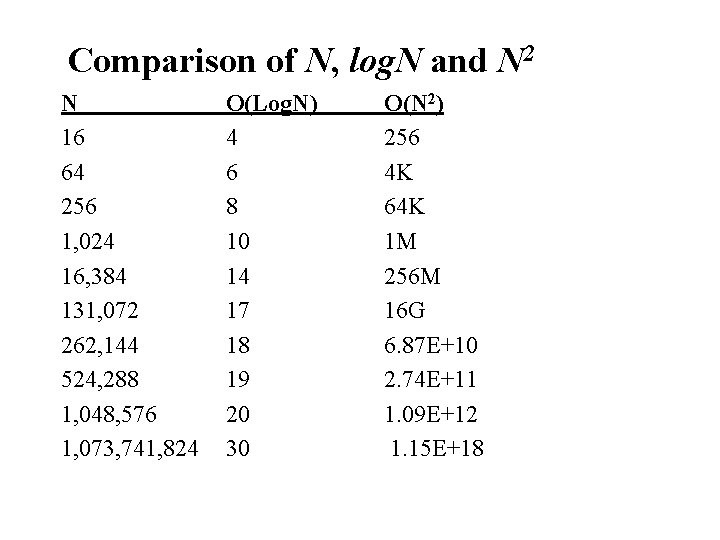Comparison of N, log. N and N 2 N 16 64 256 1, 024 16, 384 131, 072 262, 144 524, 288 1, 048, 576 1, 073, 741, 824 O(Log. N) 4 6 8 10 14 17 18 19 20 30 O(N 2) 256 4 K 64 K 1 M 256 M 16 G 6. 87 E+10 2. 74 E+11 1. 09 E+12 1. 15 E+18Insertion Sort • Insertion sort is a simple sorting algorithm that is appropriate for small inputs. – Most common sorting technique used by card players. • The list is divided into two parts: sorted and unsorted. • In each pass, the first element of the unsorted part is picked up, transferred to the sorted sublist, and inserted at the appropriate place. • A list of n elements will take at most n-1 passes to sort the data.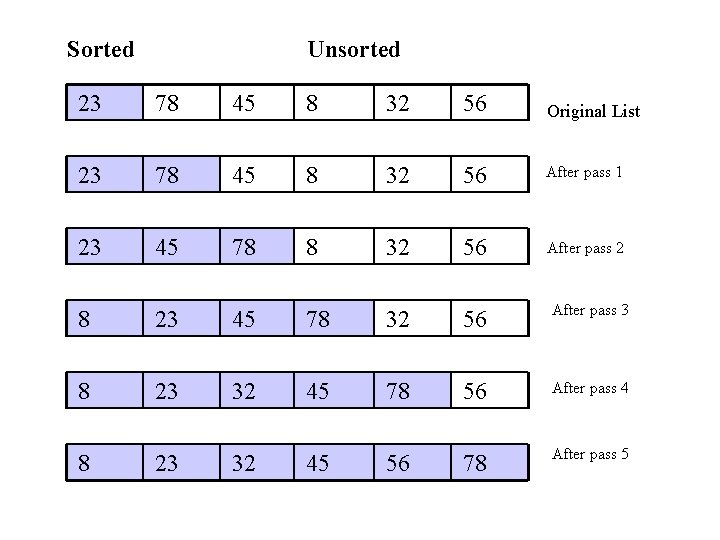Sorted Unsorted 23 78 45 8 32 56 Original List 23 78 45 8 32 56 After pass 1 23 45 78 8 32 56 After pass 2 8 23 45 78 32 56 After pass 3 8 23 32 45 78 56 After pass 4 8 23 32 45 56 78 After pass 5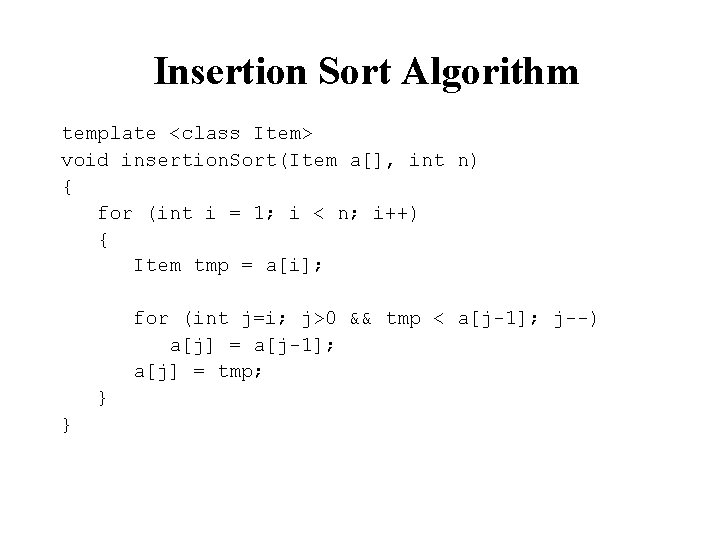Insertion Sort Algorithm template <class Item> void insertion. Sort(Item a[], int n) { for (int i = 1; i < n; i++) { Item tmp = a[i]; for (int j=i; j>0 && tmp < a[j-1]; j--) a[j] = a[j-1]; a[j] = tmp; } }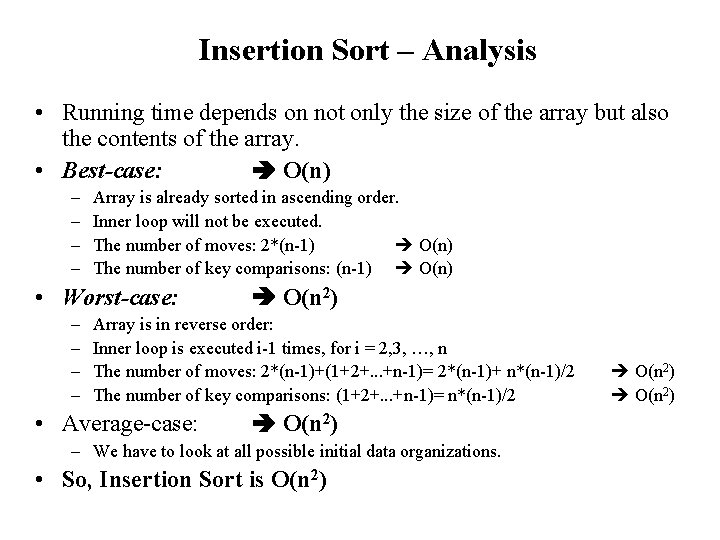Insertion Sort – Analysis • Running time depends on not only the size of the array but also the contents of the array. • Best-case: O(n) – – Array is already sorted in ascending order. Inner loop will not be executed. The number of moves: 2*(n-1) O(n) The number of key comparisons: (n-1) O(n) • Worst-case: – – O(n 2) Array is in reverse order: Inner loop is executed i-1 times, for i = 2, 3, …, n The number of moves: 2*(n-1)+(1+2+. . . +n-1)= 2*(n-1)+ n*(n-1)/2 The number of key comparisons: (1+2+. . . +n-1)= n*(n-1)/2 • Average-case: O(n 2) – We have to look at all possible initial data organizations. • So, Insertion Sort is O(n 2)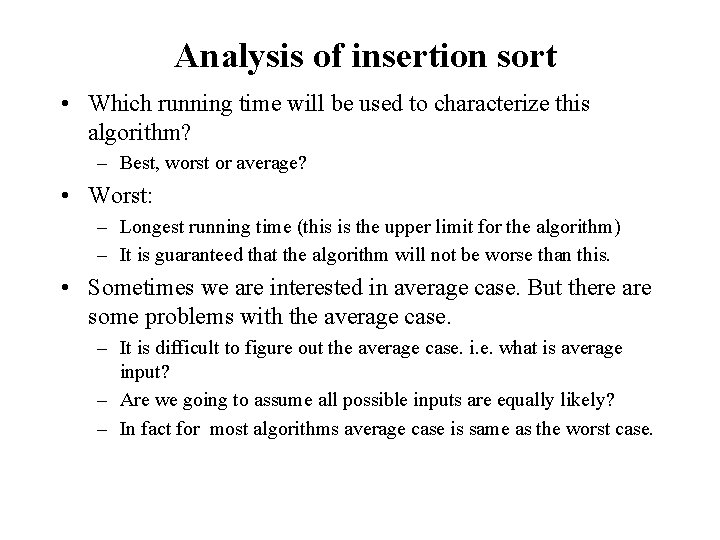Analysis of insertion sort • Which running time will be used to characterize this algorithm? – Best, worst or average? • Worst: – Longest running time (this is the upper limit for the algorithm) – It is guaranteed that the algorithm will not be worse than this. • Sometimes we are interested in average case. But there are some problems with the average case. – It is difficult to figure out the average case. i. e. what is average input? – Are we going to assume all possible inputs are equally likely? – In fact for most algorithms average case is same as the worst case.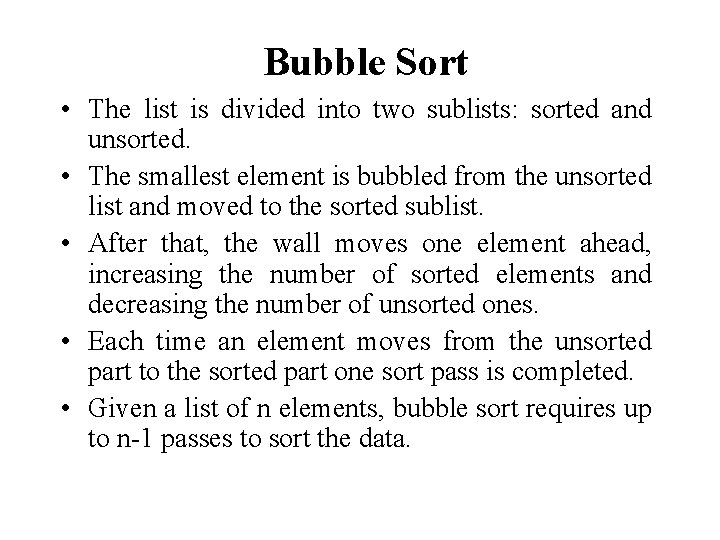Bubble Sort • The list is divided into two sublists: sorted and unsorted. • The smallest element is bubbled from the unsorted list and moved to the sorted sublist. • After that, the wall moves one element ahead, increasing the number of sorted elements and decreasing the number of unsorted ones. • Each time an element moves from the unsorted part to the sorted part one sort pass is completed. • Given a list of n elements, bubble sort requires up to n-1 passes to sort the data.Bubble Sort 23 78 45 8 32 56 Original List 8 23 78 45 32 56 After pass 1 8 23 32 78 45 56 After pass 2 8 23 32 45 78 56 After pass 3 8 23 32 45 56 78 After pass 4Bubble Sort Algorithm template <class Item> void buble. Sort(Item a[], int n) { bool sorted = false; int last = n-1; for (int i = 0; (i < last) && !sorted; i++){ sorted = true; for (int j=last; j > i; j--) if (a[j-1] > a[j]{ swap(a[j], a[j-1]); sorted = false; // signal exchange } } }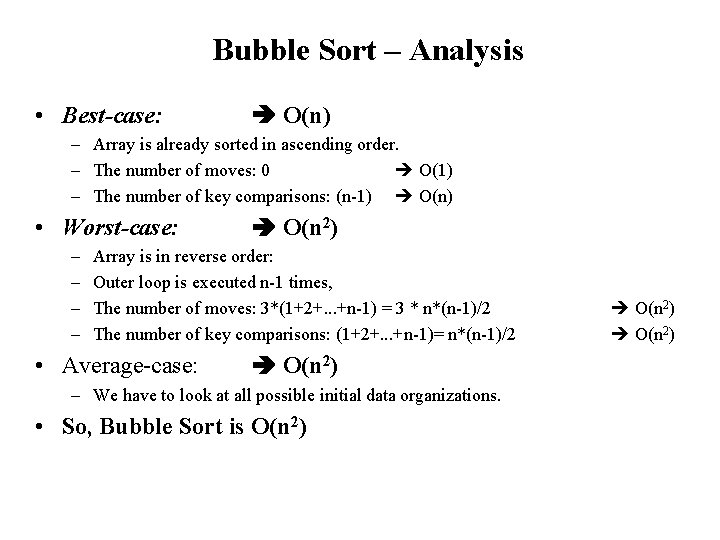Bubble Sort – Analysis • Best-case: O(n) – Array is already sorted in ascending order. – The number of moves: 0 O(1) – The number of key comparisons: (n-1) O(n) • Worst-case: – – O(n 2) Array is in reverse order: Outer loop is executed n-1 times, The number of moves: 3*(1+2+. . . +n-1) = 3 * n*(n-1)/2 The number of key comparisons: (1+2+. . . +n-1)= n*(n-1)/2 • Average-case: O(n 2) – We have to look at all possible initial data organizations. • So, Bubble Sort is O(n 2)Mergesort • Mergesort algorithm is one of two important divide-and-conquer sorting algorithms (the other one is quicksort). • It is a recursive algorithm. – Divides the list into halves, – Sort each halve separately, and – Then merge the sorted halves into one sorted array.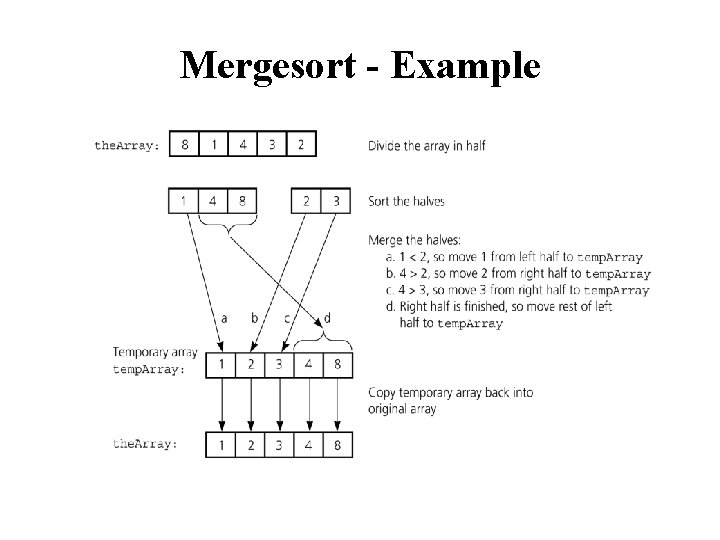Mergesort - Example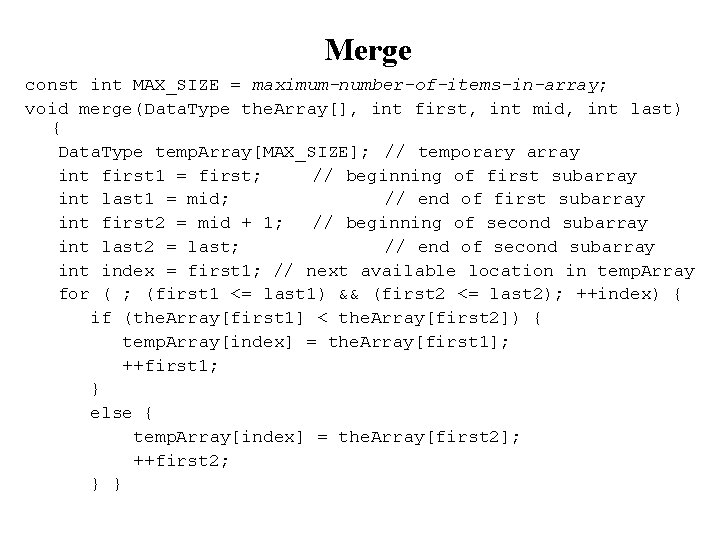Merge const int MAX_SIZE = maximum-number-of-items-in-array; void merge(Data. Type the. Array[], int first, int mid, int last) { Data. Type temp. Array[MAX_SIZE]; // temporary array int first 1 = first; // beginning of first subarray int last 1 = mid; // end of first subarray int first 2 = mid + 1; // beginning of second subarray int last 2 = last; // end of second subarray int index = first 1; // next available location in temp. Array for ( ; (first 1 <= last 1) && (first 2 <= last 2); ++index) { if (the. Array[first 1] < the. Array[first 2]) { temp. Array[index] = the. Array[first 1]; ++first 1; } else { temp. Array[index] = the. Array[first 2]; ++first 2; } }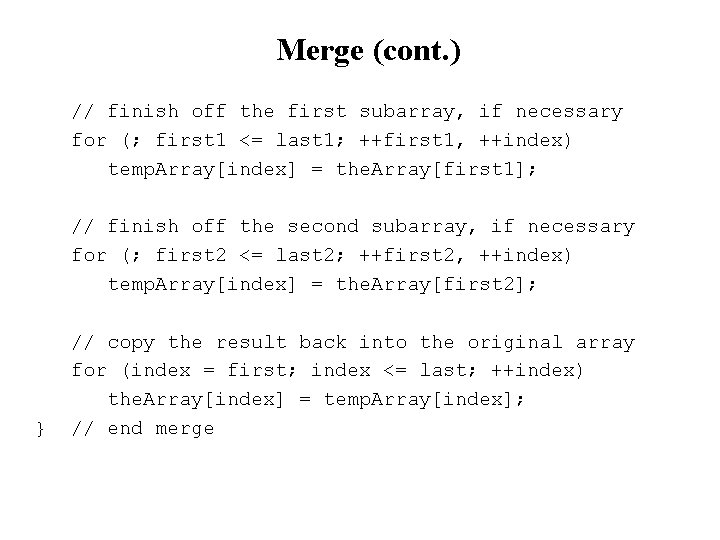Merge (cont. ) // finish off the first subarray, if necessary for (; first 1 <= last 1; ++first 1, ++index) temp. Array[index] = the. Array[first 1]; // finish off the second subarray, if necessary for (; first 2 <= last 2; ++first 2, ++index) temp. Array[index] = the. Array[first 2]; } // copy the result back into the original array for (index = first; index <= last; ++index) the. Array[index] = temp. Array[index]; // end merge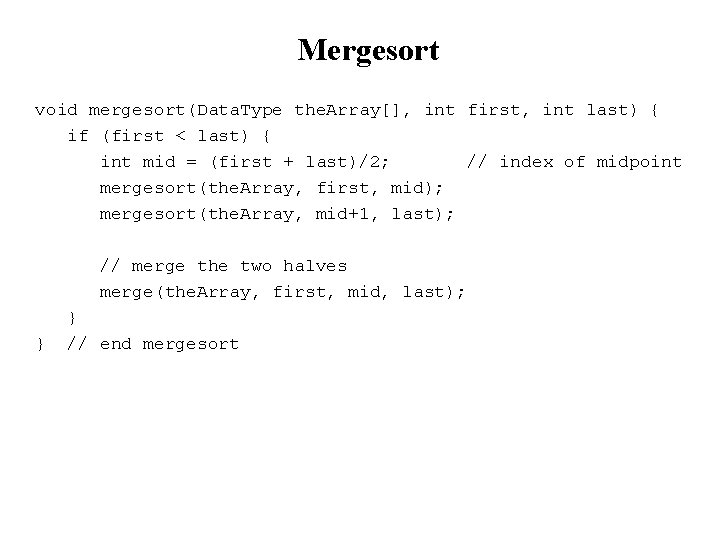Mergesort void mergesort(Data. Type the. Array[], int first, int last) { if (first < last) { int mid = (first + last)/2; // index of midpoint mergesort(the. Array, first, mid); mergesort(the. Array, mid+1, last); // merge the two halves merge(the. Array, first, mid, last); } } // end mergesortMergesort - Example 6 3 9 divide 15472 6 3 9 1 5 divide 472 divide 6 3 9 1 divide 6 3 3 6 54 1 5 merge 1 3 6 9 divide 9 merge 7 2 4 7 merge 1 9 45 merge 12345789 2 merge 2 7 merge 2457Mergesort – Example 2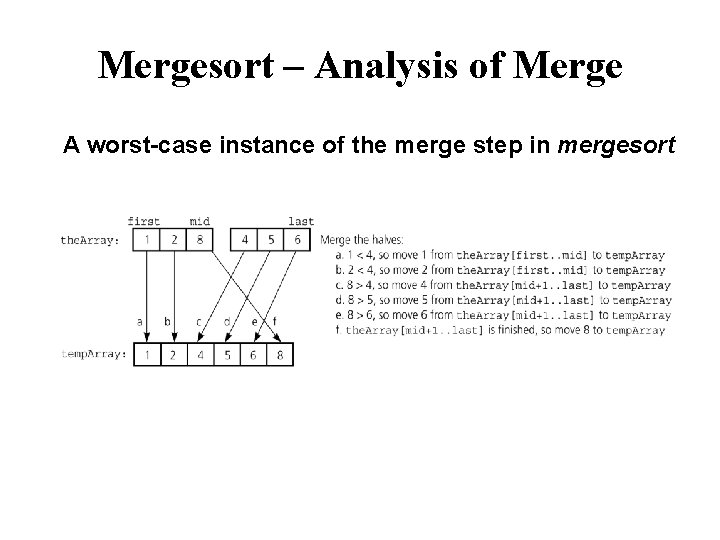Mergesort – Analysis of Merge A worst-case instance of the merge step in mergesortMergesort – Analysis of Merge (cont. ) Merging two sorted arrays of size k 0 k-1 . . . 0 2 k-1 • Best-case: . . . – All the elements in the first array are smaller (or larger) than all the elements in the second array. – The number of moves: 2 k + 2 k – The number of key comparisons: k • Worst-case: – The number of moves: 2 k + 2 k – The number of key comparisons: 2 k-1Mergesort - Analysis Levels of recursive calls to mergesort, given an array of eight items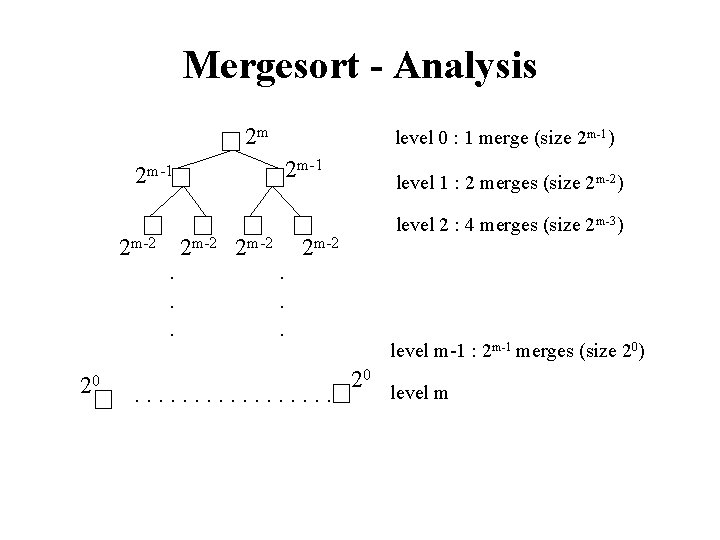Mergesort - Analysis 2 m 2 m-1 level 0 : 1 merge (size 2 m-1) 2 m-1 level 1 : 2 merges (size 2 m-2) level 2 : 4 merges (size 2 m-3) 2 m-2. . . 20 . . . . level m-1 : 2 m-1 merges (size 20) 20 level m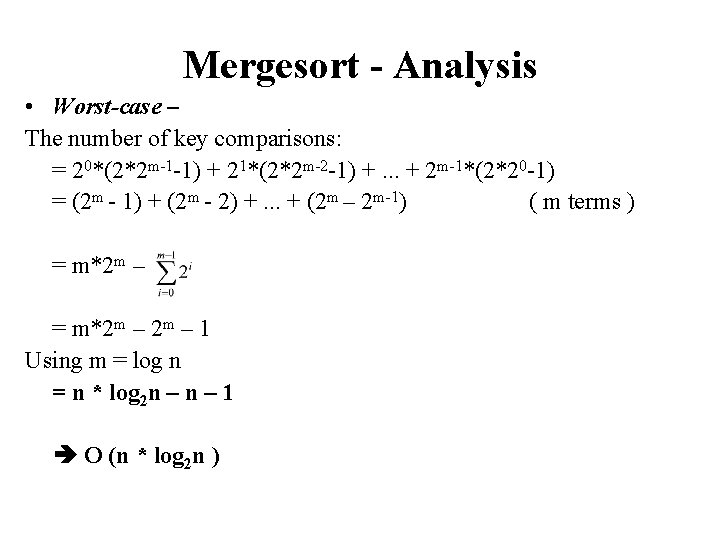Mergesort - Analysis • Worst-case – The number of key comparisons: = 20*(2*2 m-1 -1) + 21*(2*2 m-2 -1) +. . . + 2 m-1*(2*20 -1) = (2 m - 1) + (2 m - 2) +. . . + (2 m – 2 m-1) ( m terms ) = m*2 m – 1 Using m = log n = n * log 2 n – 1 O (n * log 2 n )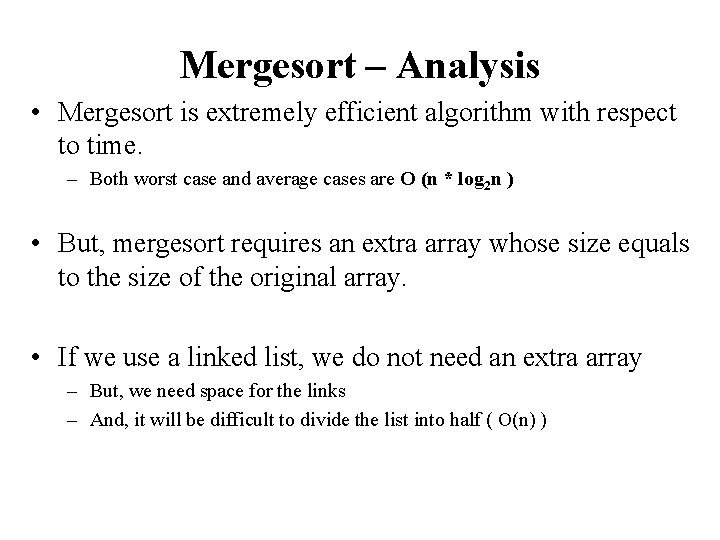Mergesort – Analysis • Mergesort is extremely efficient algorithm with respect to time. – Both worst case and average cases are O (n * log 2 n ) • But, mergesort requires an extra array whose size equals to the size of the original array. • If we use a linked list, we do not need an extra array – But, we need space for the links – And, it will be difficult to divide the list into half ( O(n) )Quicksort • • • Like mergesort, Quicksort is also based on the divide-and-conquer paradigm. But it uses this technique in a somewhat opposite manner, as all the hard work is done before the recursive calls. It works as follows: 1. First, it partitions an array into two parts, 2. Then, it sorts the parts independently, 3. Finally, it combines the sorted subsequences by a simple concatenation.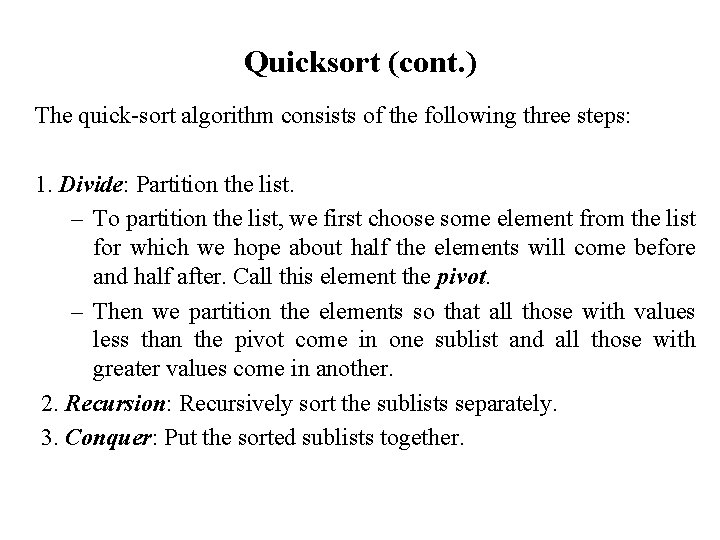Quicksort (cont. ) The quick-sort algorithm consists of the following three steps: 1. Divide: Partition the list. – To partition the list, we first choose some element from the list for which we hope about half the elements will come before and half after. Call this element the pivot. – Then we partition the elements so that all those with values less than the pivot come in one sublist and all those with greater values come in another. 2. Recursion: Recursively sort the sublists separately. 3. Conquer: Put the sorted sublists together.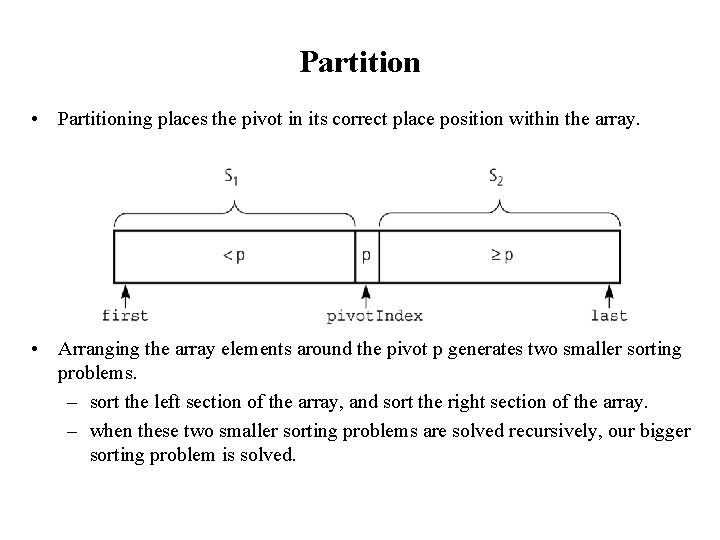Partition • Partitioning places the pivot in its correct place position within the array. • Arranging the array elements around the pivot p generates two smaller sorting problems. – sort the left section of the array, and sort the right section of the array. – when these two smaller sorting problems are solved recursively, our bigger sorting problem is solved.Partition – Choosing the pivot • First, we have to select a pivot element among the elements of the given array, and we put this pivot into the first location of the array before partitioning. • Which array item should be selected as pivot? – Somehow we have to select a pivot, and we hope that we will get a good partitioning. – If the items in the array arranged randomly, we choose a pivot randomly. – We can choose the first or last element as a pivot (it may not give a good partitioning). – We can use different techniques to select the pivot.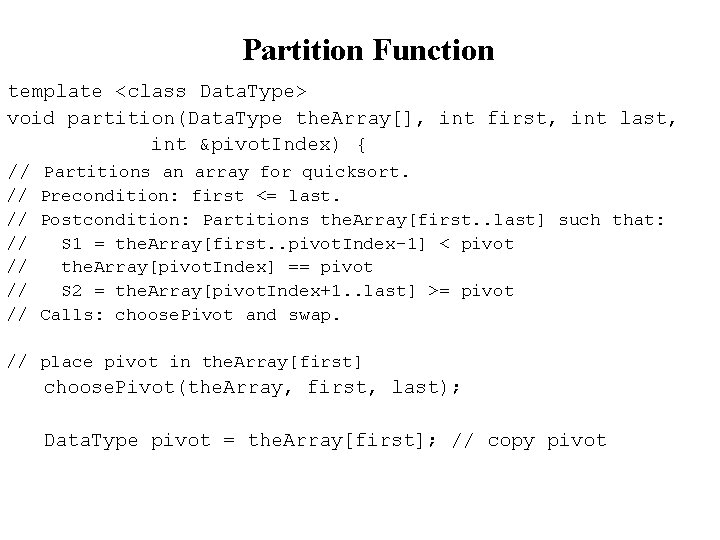Partition Function template <class Data. Type> void partition(Data. Type the. Array[], int first, int last, int &pivot. Index) { // Partitions an array for quicksort. // Precondition: first <= last. // Postcondition: Partitions the. Array[first. . last] such that: // S 1 = the. Array[first. . pivot. Index-1] < pivot // the. Array[pivot. Index] == pivot // S 2 = the. Array[pivot. Index+1. . last] >= pivot // Calls: choose. Pivot and swap. // place pivot in the. Array[first] choose. Pivot(the. Array, first, last); Data. Type pivot = the. Array[first]; // copy pivotPartition Function (cont. ) // initially, everything but pivot is in unknown int last. S 1 = first; // index of last item in S 1 int first. Unknown = first + 1; //index of 1 st item in unknown // move one item at a time until unknown region is empty for (; first. Unknown <= last; ++first. Unknown) { // Invariant: the. Array[first+1. . last. S 1] < pivot // the. Array[last. S 1+1. . first. Unknown-1] >= pivot // move item from unknown to proper region if (the. Array[first. Unknown] < pivot) { // belongs to S 1 ++last. S 1; swap(the. Array[first. Unknown], the. Array[last. S 1]); } // else belongs to S 2 } // place pivot in proper position and mark its location swap(the. Array[first], the. Array[last. S 1]); pivot. Index = last. S 1; } // end partition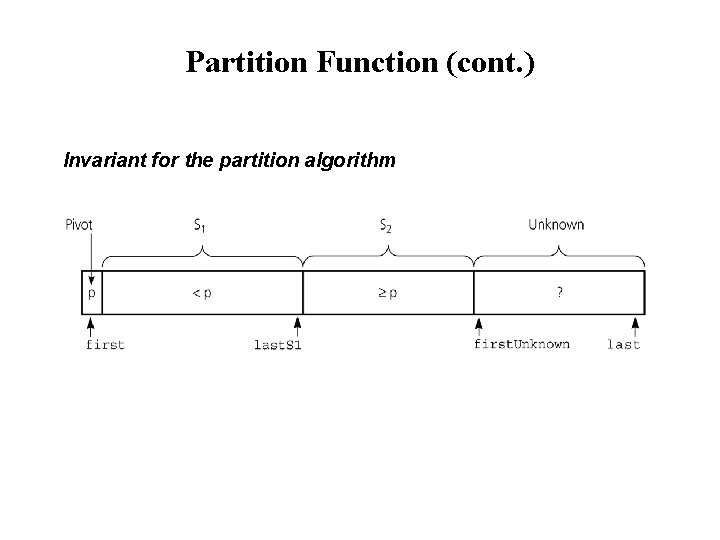Partition Function (cont. ) Invariant for the partition algorithm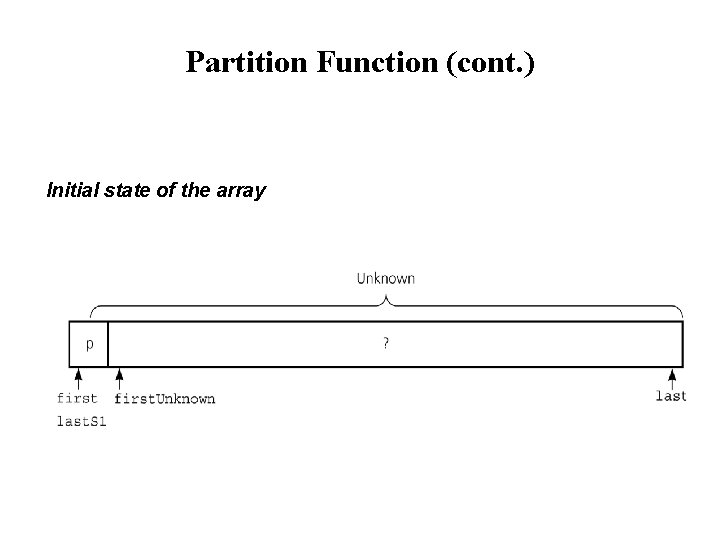Partition Function (cont. ) Initial state of the array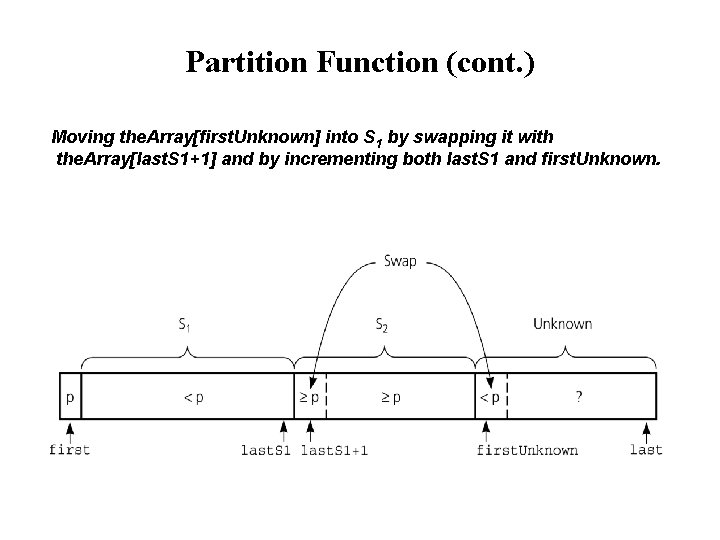Partition Function (cont. ) Moving the. Array[first. Unknown] into S 1 by swapping it with the. Array[last. S 1+1] and by incrementing both last. S 1 and first. Unknown.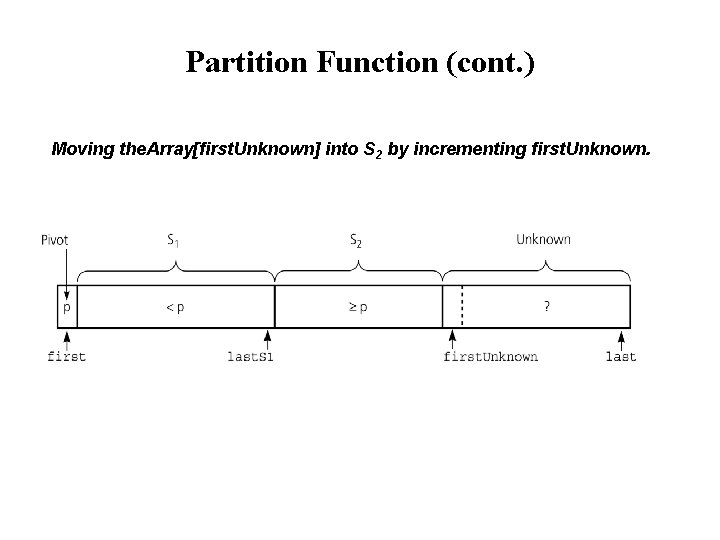Partition Function (cont. ) Moving the. Array[first. Unknown] into S 2 by incrementing first. Unknown.Partition Function (cont. ) Developing the first partition of an array when the pivot is the first itemQuicksort Function void quicksort(Data. Type the. Array[], int first, int last) { // Sorts the items in an array into ascending order. // Precondition: the. Array[first. . last] is an array. // Postcondition: the. Array[first. . last] is sorted. // Calls: partition. int pivot. Index; if (first < last) { // create the partition: S 1, pivot, S 2 partition(the. Array, first, last, pivot. Index); // sort regions S 1 and S 2 quicksort(the. Array, first, pivot. Index-1); quicksort(the. Array, pivot. Index+1, last); } }Quicksort – Analysis Worst Case: (assume that we are selecting the first element as pivot) – The pivot divides the list of size n into two sublists of sizes 0 and n-1. – The number of key comparisons = n-1 + n-2 +. . . + 1 = n 2/2 – n/2 O(n 2) – The number of swaps = = n-1 + n-2 +. . . + 1 swaps outside of the for loop = n 2/2 + n/2 - 1 O(n 2) • So, Quicksort is O(n 2) in worst case swaps inside of the for loopQuicksort – Analysis • Quicksort is O(n*log 2 n) in the best case and average case. • Quicksort is slow when the array is sorted and we choose the first element as the pivot. • Although the worst case behavior is not so good, its average case behavior is much better than its worst case. – So, Quicksort is one of best sorting algorithms using key comparisons.Quicksort – Analysis A worst-case partitioning with quicksortQuicksort – Analysis An average-case partitioning with quicksort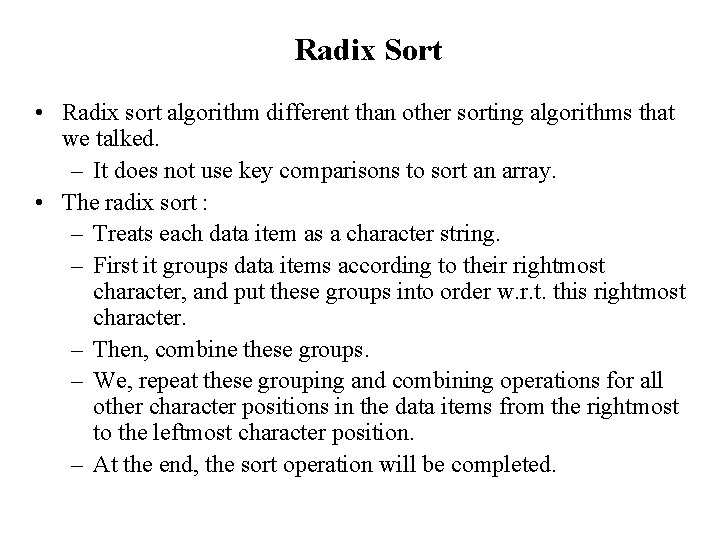Radix Sort • Radix sort algorithm different than other sorting algorithms that we talked. – It does not use key comparisons to sort an array. • The radix sort : – Treats each data item as a character string. – First it groups data items according to their rightmost character, and put these groups into order w. r. t. this rightmost character. – Then, combine these groups. – We, repeat these grouping and combining operations for all other character positions in the data items from the rightmost to the leftmost character position. – At the end, the sort operation will be completed.